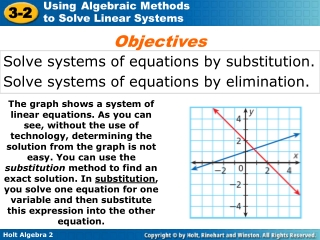DownloadDownload PresentationSolve systems of equations by substitution. Solve systems of equations by elimination.

# Solve systems of equations by substitution. Solve systems of equations by elimination.

Télécharger la présentation## Solve systems of equations by substitution. Solve systems of equations by elimination.

- - - - - - - - - - - - - - - - - - - - - - - - - - - E N D - - - - - - - - - - - - - - - - - - - - - - - - - - -
##### Presentation Transcript

1. Objectives Solve systems of equations by substitution. Solve systems of equations by elimination. The graph shows a system of linear equations. As you can see, without the use of technology, determining the solution from the graph is not easy. You can use the substitution method to find an exact solution. In substitution, you solve one equation for one variable and then substitute this expression into the other equation.

2. y= x – 1 x + y = 7 Use substitution to solve the system of equations.

3. y= 2x – 1 3x + 2y = 26 Use substitution to solve the system of equations.

4. y= 4x –9 y = -2x + 3 Use substitution to solve the system of equations.

5. 2y + x= 4 3x– 4y = 7 Use substitution to solve the system of equations.

6. 5x + 6y= –9 2x– 2 = –y Use substitution to solve the system of equations.

7. Reading Math The elimination method is sometimes called the addition method or linear combination. You can also solve systems of equations with the elimination method. With elimination, you get rid of one of the variables by adding or subtracting equations. You may have to multiply one or both equations by a number to create variable terms that can be eliminated.

8. 3x + 2y = 4 4x– 2y = –18 Use elimination to solve the system of equations.

9. Use elimination to solve the system of equations. 4x + 7y= –25 –12x–7y = 19

10. 2x – 3y = -5 -4x+ 2y = -10 Use elimination to solve the system of equations.

11. 3x + 5y = –16 2x+ 3y = –9 Use elimination to solve the system of equations.

12. 5x – 3y = 42 8x+ 5y = 28 Use elimination to solve the system of equations.

13. Remember! An identity, such as 0 = 0, is always true and indicates infinitely many solutions. A contradiction, such as 1 = 3, is never true and indicates no solution. In Lesson 3–1, you learned that systems may have infinitely many or no solutions. When you try to solve these systems algebraically, the result will be an identity or a contradiction.

14. Classify the system and determine the number of solutions. 3x + y = 1 2y+ 6x = –18

15. Classify the system and determine the number of solutions. 56x + 8y = –32 7x + y = –4

16. Classify the system and determine the number of solutions. 6x + 3y = –12 2x+ y = –6